# 摄像机

## 摄像机/观察空间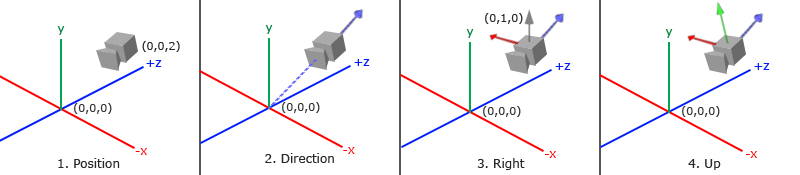### 1. 摄像机位置

glm::vec3 cameraPos = glm::vec3(0.0f, 0.0f, 3.0f);


Important

### 2. 摄像机方向

glm::vec3 cameraTarget = glm::vec3(0.0f, 0.0f, 0.0f);
glm::vec3 cameraDirection = glm::normalize(cameraPos - cameraTarget);


Attention

### 3. 右轴

glm::vec3 up = glm::vec3(0.0f, 1.0f, 0.0f);
glm::vec3 cameraRight = glm::normalize(glm::cross(up, cameraDirection));


### 4. 上轴

glm::vec3 cameraUp = glm::cross(cameraDirection, cameraRight);


## Look At

glm::mat4 view;
view = glm::lookAt(glm::vec3(0.0f, 0.0f, 3.0f),
glm::vec3(0.0f, 0.0f, 0.0f),
glm::vec3(0.0f, 1.0f, 0.0f));


glm::LookAt函数需要一个位置、目标和上向量。它会创建一个和在上一节使用的一样的观察矩阵。

float radius = 10.0f;
float camX = sin(glfwGetTime()) * radius;
float camZ = cos(glfwGetTime()) * radius;
glm::mat4 view;
view = glm::lookAt(glm::vec3(camX, 0.0, camZ), glm::vec3(0.0, 0.0, 0.0), glm::vec3(0.0, 1.0, 0.0));


# 自由移动

glm::vec3 cameraPos   = glm::vec3(0.0f, 0.0f,  3.0f);
glm::vec3 cameraFront = glm::vec3(0.0f, 0.0f, -1.0f);
glm::vec3 cameraUp    = glm::vec3(0.0f, 1.0f,  0.0f);


LookAt函数现在成了：

view = glm::lookAt(cameraPos, cameraPos + cameraFront, cameraUp);


void processInput(GLFWwindow *window)
{
...
float cameraSpeed = 0.05f; // adjust accordingly
if (glfwGetKey(window, GLFW_KEY_W) == GLFW_PRESS)
cameraPos += cameraSpeed * cameraFront;
if (glfwGetKey(window, GLFW_KEY_S) == GLFW_PRESS)
cameraPos -= cameraSpeed * cameraFront;
if (glfwGetKey(window, GLFW_KEY_A) == GLFW_PRESS)
cameraPos -= glm::normalize(glm::cross(cameraFront, cameraUp)) * cameraSpeed;
if (glfwGetKey(window, GLFW_KEY_D) == GLFW_PRESS)
cameraPos += glm::normalize(glm::cross(cameraFront, cameraUp)) * cameraSpeed;
}


Important

## 移动速度

float deltaTime = 0.0f; // 当前帧与上一帧的时间差
float lastFrame = 0.0f; // 上一帧的时间


float currentFrame = glfwGetTime();
deltaTime = currentFrame - lastFrame;
lastFrame = currentFrame;


void processInput(GLFWwindow *window)
{
float cameraSpeed = 2.5f * deltaTime;
...
}


# 视角移动

## 欧拉角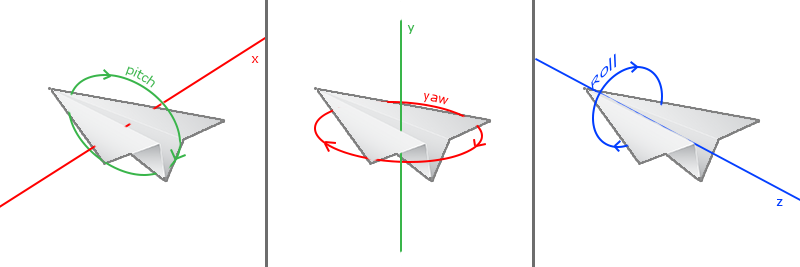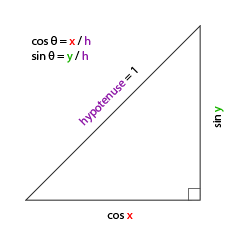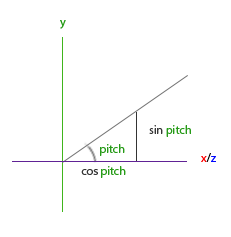direction.y = sin(glm::radians(pitch)); // 注意我们先把角度转为弧度


direction.x = cos(glm::radians(pitch));
direction.z = cos(glm::radians(pitch));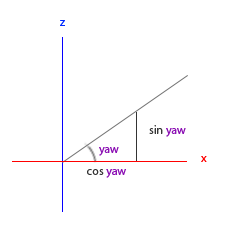direction.x = cos(glm::radians(pitch)) * cos(glm::radians(yaw)); // 译注：direction代表摄像机的前轴(Front)，这个前轴是和本文第一幅图片的第二个摄像机的方向向量是相反的
direction.y = sin(glm::radians(pitch));
direction.z = cos(glm::radians(pitch)) * sin(glm::radians(yaw));


## 鼠标输入

glfwSetInputMode(window, GLFW_CURSOR, GLFW_CURSOR_DISABLED);


void mouse_callback(GLFWwindow* window, double xpos, double ypos);


glfwSetCursorPosCallback(window, mouse_callback);


1. 计算鼠标距上一帧的偏移量。
2. 把偏移量添加到摄像机的俯仰角和偏航角中。
3. 对偏航角和俯仰角进行最大和最小值的限制。
4. 计算方向向量。

float lastX = 400, lastY = 300;


float xoffset = xpos - lastX;
float yoffset = lastY - ypos; // 注意这里是相反的，因为y坐标是从底部往顶部依次增大的
lastX = xpos;
lastY = ypos;

float sensitivity = 0.05f;
xoffset *= sensitivity;
yoffset *= sensitivity;


yaw   += xoffset;
pitch += yoffset;


if(pitch > 89.0f)
pitch =  89.0f;
if(pitch < -89.0f)
pitch = -89.0f;


glm::vec3 front;
front.x = cos(glm::radians(pitch)) * cos(glm::radians(yaw));
front.y = sin(glm::radians(pitch));
front.z = cos(glm::radians(pitch)) * sin(glm::radians(yaw));
cameraFront = glm::normalize(front);


if(firstMouse) // 这个bool变量初始时是设定为true的
{
lastX = xpos;
lastY = ypos;
firstMouse = false;
}


void mouse_callback(GLFWwindow* window, double xpos, double ypos)
{
if(firstMouse)
{
lastX = xpos;
lastY = ypos;
firstMouse = false;
}

float xoffset = xpos - lastX;
float yoffset = lastY - ypos;
lastX = xpos;
lastY = ypos;

float sensitivity = 0.05;
xoffset *= sensitivity;
yoffset *= sensitivity;

yaw   += xoffset;
pitch += yoffset;

if(pitch > 89.0f)
pitch = 89.0f;
if(pitch < -89.0f)
pitch = -89.0f;

glm::vec3 front;
front.x = cos(glm::radians(yaw)) * cos(glm::radians(pitch));
front.y = sin(glm::radians(pitch));
front.z = sin(glm::radians(yaw)) * cos(glm::radians(pitch));
cameraFront = glm::normalize(front);
}


## 缩放

void scroll_callback(GLFWwindow* window, double xoffset, double yoffset)
{
if(fov >= 1.0f && fov <= 45.0f)
fov -= yoffset;
if(fov <= 1.0f)
fov = 1.0f;
if(fov >= 45.0f)
fov = 45.0f;
}


projection = glm::perspective(glm::radians(fov), 800.0f / 600.0f, 0.1f, 100.0f);


glfwSetScrollCallback(window, scroll_callback);


Important

# 摄像机类

Attention

## 练习

• 看看你是否能够修改摄像机类，使得其能够变成一个真正的FPS摄像机（也就是说不能够随意飞行）；你只能够呆在xz平面上：参考解答

• 试着创建你自己的LookAt函数，其中你需要手动创建一个我们在一开始讨论的观察矩阵。用你的函数实现来替换GLM的LookAt函数，看看它是否还能一样地工作：参考解答